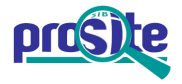Entry: PS00539

 Entry name [info] PYROKININ Accession [info] PS00539 Entry type [info] PATTERN Date [info] 01-DEC-1991 CREATED; 01-JUN-1992 DATA UPDATE; 02-JUN-2021 INFO UPDATE. PROSITE Doc. [info] PDOC00466

Name and characterization of the entry

 Description [info] Pyrokinins signature. Pattern [info] ```F-[GSTV]-P-R-L-[G>]. ```

Numerical results [info]

Numerical results for UniProtKB/Swiss-Prot release 2021_03 which contains 565'254 sequence entries.

 Total number of hits 167 in 144 different sequences Number of true positive hits 144 in 124 different sequences Number of 'unknown' hits 4 in 1 sequence Number of false positive hits 19 in 19 different sequences Number of false negative sequences 23 Number of 'partial' sequences 0 Precision (true positives / (true positives + false positives)) 88.34 % Recall (true positives / (true positives + false negatives)) 86.23 %

 UniProtKB/Swiss-ProtTrue positive sequences 124 sequences ```CAPA_AGRIP (C0HKS6 ), CP2B_DROME (Q9NIP6 ), CP2B_DROPS (Q29CA0 ), ``` ```LMT1_LOCMI (P22395 ), LMT2_LOCMI (P22396 ), LMT3_LOCMI (P41489 ), LMT4_LOCMI (P41490 ), LPK1_LOCMI (P20404 ), LPK2_LOCMI (P41488 ), LPK_RHYMA (P13049 ), PBAN_AEDAE (Q16N80 ), PBAN_AGRIP (O76818 ), PBAN_ANOGA (Q7PTL2 ), PBAN_APIME (A8CL69 ), PBAN_BOMMO (P09971 ), PBAN_HELAU (O18641 ), PBAN_HELZE (P11159 ), PBAN_LYMDI (P43511 ), PBAN_MAMBR (O45027 ), PBAN_SPOLI (Q95P48 ), PHPT_MYTSE (P25271 ), PK1_DELRA (B3EWL1 ), PPK3_AUSGA (B3A0F1 ), PPK3_AUSRA (B3A0B3 ), PPK3_HEMMO (B3A0D2 ), PPK3_KARBI (B3A074 ), PPK3_KARBO (B3A055 ), PPK3_LOBRE (B3A093 ), PPK3_NAMOO (B0M2U4 ), PPK3_STRNA (B0M3B9 ), PPK4_AUSGA (B3A0D7 ), PPK4_AUSRA (B3A098 ), PPK4_HEMMO (B3A0B8 ), PPK4_KARBI (B3A060 ), PPK4_KARBO (B0M3E5 ), PPK4_LOBRE (B3A079 ), PPK4_MANKU (B0M3C4 ), PPK4_NAMOO (P86996 ), PPK4_STRNA (B0M398 ), PPK5A_BLADU (P84594 ), PPK5A_PYCSU (P84667 ), PPK5B_BLADU (P85548 ), PPK5B_PYCSU (P84668 ), PPK5_APTFU (P84666 ), PPK5_ARCTE (P84595 ), PPK5_BANRO (P85542 ), PPK5_BLACR (P85545 ), PPK5_BLAGE (P85554 ), PPK5_BLAGI (P85556 ), PPK5_BLAOR (P85559 ), PPK5_BLEDI (P85564 ), PPK5_CRYDW (P85569 ), PPK5_CRYKY (P85574 ), PPK5_CYRPO (P85579 ), PPK5_DERAT (P85596 ), PPK5_DERCR (P84672 ), PPK5_DERER (P85584 ), PPK5_DERIN (P85590 ), PPK5_DERVE (P84671 ), PPK5_DIPPU (P84665 ), PPK5_ELLSS (P85607 ), PPK5_ERGCA (P85611 ), PPK5_EUBDI (P85617 ), PPK5_EUBPO (P85623 ), PPK5_EUBSB (P85629 ), PPK5_EURFL (P85633 ), PPK5_GROGR (P85638 ), PPK5_GROPO (P85641 ), PPK5_GYNCS (P85646 ), PPK5_GYNLU (P85650 ), PPK5_HOSCA (P85796 ), PPK5_LAXSP (P84669 ), PPK5_LAXSS (P85656 ), PPK5_LOBDE (P85659 ), PPK5_LUCGR (P85663 ), PPK5_LUCSU (P85669 ), PPK5_LUCVE (P85675 ), PPK5_MASDA (P85681 ), PPK5_NEORO (P85685 ), PPK5_PACBA (B3A0J4 ), PPK5_PANSB (P85690 ), PPK5_PANSS (P85696 ), PPK5_PANVI (P84670 ), PPK5_PERAM (P82617 ), PPK5_PERAU (P85702 ), PPK5_PERBB (P85708 ), PPK5_PERBR (P85710 ), PPK5_PERFU (P85714 ), PPK5_PERRU (P85720 ), PPK5_PERSR (P85730 ), PPK5_PERSS (P85726 ), PPK5_PERVR (P85733 ), PPK5_PILDU (P85737 ), PPK5_POLAY (P85741 ), PPK5_PRAMA (B3A0F6 ), PPK5_PRIVA (P85747 ), PPK5_PSEBJ (P85751 ), PPK5_PSEFO (P85759 ), PPK5_PSEFV (P85755 ), PPK5_RHYMA (P84664 ), PPK5_SHELA (P85773 ), PPK5_SUPDI (P85777 ), PPK5_SUPLO (P85781 ), PPK5_SYMPA (P85784 ), PPK5_THEPT (P85790 ), PPK5_TYRGL (B3A0H4 ), PPK6_BLAOR (P84420 ), PPK6_CELBM (P84362 ), PPK6_DEREJ (P84364 ), PPK6_DERER (P84363 ), PPK6_DERKE (P84365 ), PPK6_EURFL (P84370 ), PPK6_NEORO (P84361 ), PPK6_PERAM (P82693 ), PPK6_PERAU (P84368 ), PPK6_PERBR (P84359 ), PPK6_PERFU (P84360 ), PPK6_PSEBJ (P84367 ), PPK6_PSEFO (P84371 ), PPK6_PSEFV (P84366 ), PPK6_SHELA (P84421 ), PPK_CAMFO (E2AFK9 ), PPK_MUSDO (P84357 ), PPK_SARBU (P84356 ) ``` » more Retrieve an alignment of UniProtKB/Swiss-Prot true positive hits: [Clustal format, color, condensed view] [Clustal format, color] [Clustal format, plain text] [Fasta format] Retrieve the sequence logo from the alignment UniProtKB/Swiss-ProtFalse negative sequences 23 sequences ```HUGIN_DROME (Q9VG55 ), PPK1_CARMO (P82684 ), PPK1_PERAM (P82691 ), ``` ```PPK2_PERAM (P82692 ), PPK3_PERAM (P82618 ), PPK4_BLAOR (P84410 ), PPK4_CELBM (P84369 ), PPK4_DEREJ (P84415 ), PPK4_DERER (P84414 ), PPK4_DERKE (P84416 ), PPK4_EURFL (P84413 ), PPK4_NEORO (P84412 ), PPK4_PERAM (P82619 ), PPK4_PERAU (P84409 ), PPK4_PERBR (P84358 ), PPK4_PERFU (P82690 ), PPK4_PSEBJ (P84419 ), PPK4_PSEFO (P84418 ), PPK4_PSEFV (P84417 ), PPK4_SHELA (P84411 ), PPK_LOCMI (P85867 ), PPK_RHOPR (P85827 ), PPK_SCHGR (P85864 ) ``` » more UniProtKB/Swiss-Prot'Unknown' sequences 1 sequence ```PBAN_HELIE (C0HL17 ) ``` UniProtKB/Swiss-ProtFalse positive sequences 19 sequences ```ALDH2_HUMAN (P05091 ), ALDH2_PONAB (Q5RF00 ), GLGB_PSEAE (Q9I1W2 ), ``` ```LMF2_XENLA (Q7ZWN0 ), LMF2_XENTR (Q0P4Y8 ), MHPC_KLEP7 (A6TAC7 ), MHPC_PARXL (Q13QH4 ), MIAB_THEYD (B5YKW2 ), PEX5_CANAL (O74711 ), PKHA2_BOVIN (Q3ZBA3 ), RL6_BUCAI (P57576 ), RSMG_MYCBO (P59964 ), RSMG_MYCBP (A1KEG3 ), RSMG_MYCTA (A5U9P7 ), RSMG_MYCTO (P9WGW8 ), RSMG_MYCTU (P9WGW9 ), SELO_MYCPA (Q73V62 ), SELO_PSET1 (Q3IJ32 ), TRM5B_METJA (Q58293 ) ``` » more